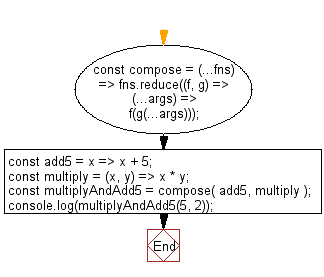# JavaScript: Perform right-to-left function composition

## JavaScript fundamental (ES6 Syntax): Exercise-66 with Solution

Write a JavaScript program to perform right-to-left function composition.

• Use Array.prototype.reduce() to perform right-to-left function composition.
• The last (rightmost) function can accept one or more arguments; the remaining functions must be unary.

Sample Solution:

JavaScript Code:

``````//#Source https://bit.ly/2neWfJ2
const compose = (...fns) => fns.reduce((f, g) => (...args) => f(g(...args)));
const add5 = x => x + 5;
const multiply = (x, y) => x * y;
multiply
);
```
```

Sample Output:

```15
```

Pictorial Presentation:Flowchart:Live Demo:

See the Pen javascript-basic-exercise-66-1 by w3resource (@w3resource) on CodePen.

Improve this sample solution and post your code through Disqus

What is the difficulty level of this exercise?

Test your Programming skills with w3resource's quiz.

﻿

## JavaScript: Tips of the Day

Chunks an array into n smaller arrays

Example:

```const tips_chunkIntoN = (arr, n) => {
const size = Math.ceil(arr.length / n);
return Array.from({ length: n }, (v, i) =>
arr.slice(i * size, i * size + size)
);
}
console.log(tips_chunkIntoN([1, 2, 3, 4, 5, 6, 7,8], 4));
```

Output:

```[[1,2],[3,4],[5,6],[7,8]]
```